# Introduction to mixed-effects modeling using the lme4 package

## Introduction

It is widely accepted that in almost any research area in the social and health sciences context plays an important role. Think of the impact of environmental stressors on the psychological health of individuals, the influence of stimulation in the environment on child development, or the effect of classrooms and schools’ characteristics on children’s education. The idea is that individuals are influenced by groups and contexts they belong. Conceptually, individuals and groups can be understood as multilevel or hierarchical systems where individuals are nested within groups. The same principle applies to other types of hierarchical structures, such as groups nested within super-groups (e.g., cities nested within countries) and repeated measures nested within individuals (e.g., longitudinal studies).

The lower level of the hierarchy is called Level-1 (L1) and the higher level of the system Level 2 (L2). For example, in a hierarchical system with children nested within schools, individuals constitute the lower level and schools the Level-2. Variables can be defined at any level and the study of those variables and their interactions is generally known as multilevel or mixed-effects modeling.

Mathematically, mixed-effects models can be seen as a hierarchical system of regression equations where L1 parameters are function of the L2 equations. The following equations represent a two-level model with one L1 predictor, X, and one L2 predictor, W.

Level-1: $$Y_{ij}=\beta_{0j}+\beta_{1j}X_{ij}+r_{ij}$$

Level-2: $$\beta_{0j}=\gamma_{00}+\gamma_{01}W_{j}+u_{0j}$$

Level-2: $$\beta_{1j}=\gamma_{10}+\gamma_{11}W_{j}+u_{1j}$$

Note that the L1 equation looks similar to an OLS regression equation, but the j subscripts remind us that $$\beta_{0j}$$ and $$\beta_{1j}$$ vary across L2 units.

Substituting and rearranging the terms in the above system of equations we obtain:

$Y_{ij}=[\gamma_{00}+\gamma_{10}X_{ij}+\gamma_{01}W_{j}+\gamma_{11}W_{j}X_{ij}]+[u_{0j}+u_{1j}X_{ij}+r_{ij}]$

This equation, even if less clearly shows the multilevel nature of the model, has an advantage: it allows us to immediately identify the fixed part and the random part of the model, that is, the gammas and the errors respectively.That’s where the the name ‘mixed-effects’ come from.

## The function and the data

To fit mixed-effects models will use the lmer function for the lme4 package. The function has the following form (look at ?lmer for more info):

lmer(dep_var ~ ind_var1 + ind_var2 + (1|L2unit), data = mydata, options)

For the examples that follow, we’ll be using the Orthodont data set from the nlme package. The Orthodont data frame has 108 rows and 4 columns of the change in an orthdontic measurement over time for several young subjects. For more info type in the R console ?Orthodont. The four variables are:

• distance A numeric vector of distances from the pituitary to the pterygomaxillary fissure (mm).
• age A numeric vector of ages of the subject (yr).
• Subject A factor indicating the subject on which the measurement was made.
• Sex A factor with two levels ‘Male’ and ‘Female’

Let’s save the data in a new dataset and look at it.

data1 <- as.data.frame(nlme::Orthodont)
data1$Subject <- factor(data1$Subject, ordered = FALSE)
head(data1)
##   distance age Subject  Sex
## 1     26.0   8     M01 Male
## 2     25.0  10     M01 Male
## 3     29.0  12     M01 Male
## 4     31.0  14     M01 Male
## 5     21.5   8     M02 Male
## 6     22.5  10     M02 Male
str(data1)
## 'data.frame':    108 obs. of  4 variables:
##  $distance: num 26 25 29 31 21.5 22.5 23 26.5 23 22.5 ... ##$ age     : num  8 10 12 14 8 10 12 14 8 10 ...
##  $Subject : Factor w/ 27 levels "M16","M05","M02",..: 15 15 15 15 3 3 3 3 7 7 ... ##$ Sex     : Factor w/ 2 levels "Male","Female": 1 1 1 1 1 1 1 1 1 1 ...

And this is how the data look like

# load libraries
library(lattice)
library(ggplot2)
library(dplyr)
##
## Attaching package: 'dplyr'
## The following objects are masked from 'package:stats':
##
##     filter, lag
## The following objects are masked from 'package:base':
##
##     intersect, setdiff, setequal, union
# line graph
data1 %>%
ggplot(aes(x=age, y=distance, group=Subject, color=Subject, linetype=Sex)) +
geom_line(size=1) + theme_classic()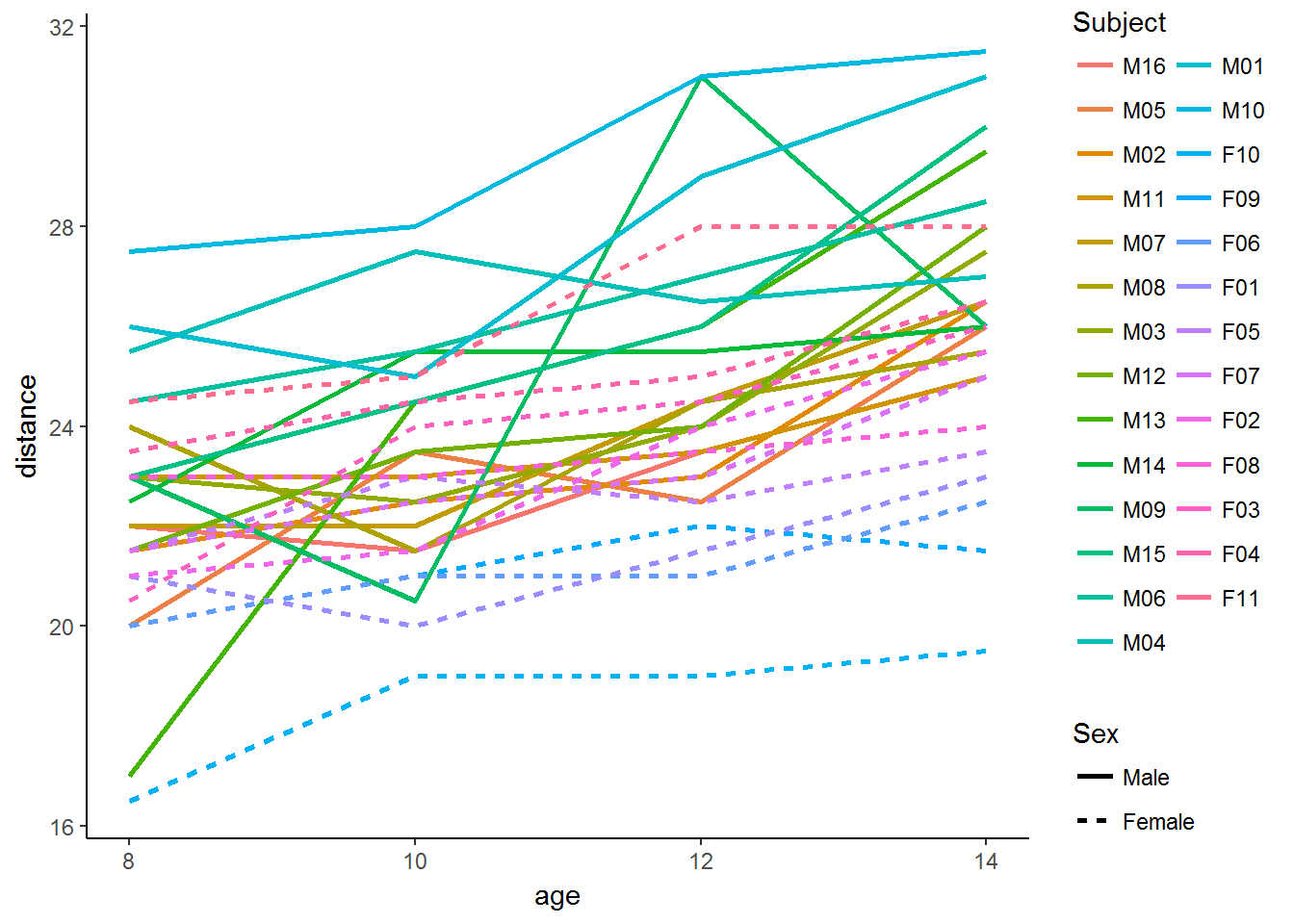## Null model

library(lme4)
## Loading required package: Matrix

Will will start by fitting a model with no predictors, a ‘null model’. The equation,

Level-1: $$distance_{ij}=\beta_{0j}+r_{ij}$$

Level-2: $$\beta_{0j}=\gamma_{00}+u_{0j}$$

and the command:

mod1 <- lmer(distance ~ (1|Subject), data=data1, REML=F)
summary(mod1)
## Linear mixed model fit by maximum likelihood  ['lmerMod']
## Formula: distance ~ (1 | Subject)
##    Data: data1
##
##      AIC      BIC   logLik deviance df.resid
##    521.5    529.5   -257.7    515.5      105
##
## Scaled residuals:
##     Min      1Q  Median      3Q     Max
## -3.2391 -0.5248 -0.1103  0.4827  2.7734
##
## Random effects:
##  Groups   Name        Variance Std.Dev.
##  Subject  (Intercept) 3.567    1.889
##  Residual             4.930    2.220
## Number of obs: 108, groups:  Subject, 27
##
## Fixed effects:
##             Estimate Std. Error t value
## (Intercept)  24.0231     0.4216   56.98

We can visualize the predicted values by plotting the outcome of the “

data1 %>%
# save predicted values
mutate(pred_dist = fitted(mod1)) %>%
# graph
ggplot(aes(x=age, y=pred_dist, group=Subject, color=Subject)) + theme_classic() +
geom_line(size=1)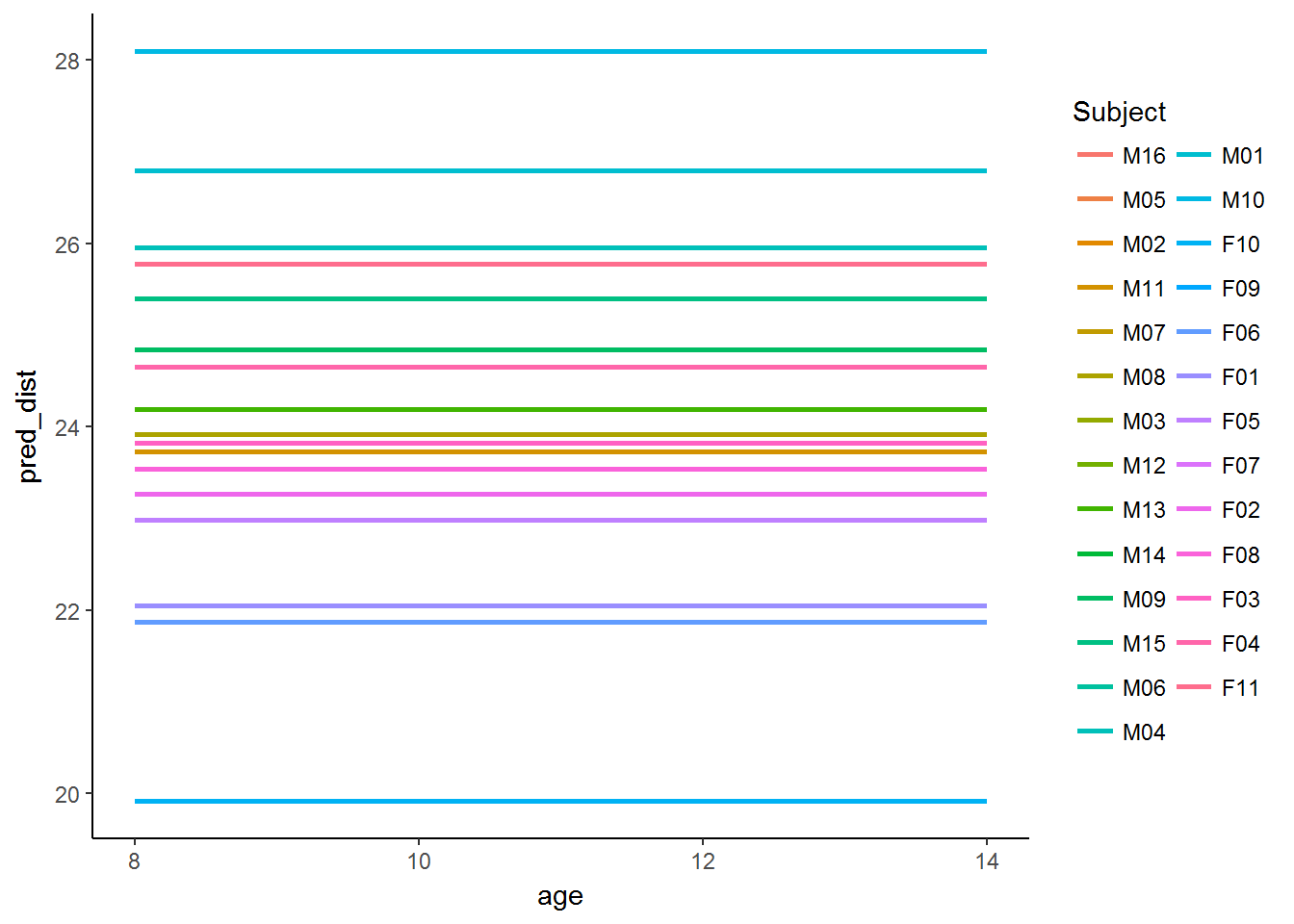## Random intercept model

In our next model we add a L1 independent variable,age.

Level-1: $$distance_{ij}=\beta_{0j}+\beta_{1j}age_{ij}+r_{ij}$$

Level-2: $$\beta_{0j}=\gamma_{00}+u_{0j}$$

Level-2: $$\beta_{1j}=\gamma_{10}$$

mod2 <- lmer(distance ~ age + (1|Subject), data=data1, REML=F)
summary(mod2)
## Linear mixed model fit by maximum likelihood  ['lmerMod']
## Formula: distance ~ age + (1 | Subject)
##    Data: data1
##
##      AIC      BIC   logLik deviance df.resid
##    451.4    462.1   -221.7    443.4      104
##
## Scaled residuals:
##     Min      1Q  Median      3Q     Max
## -3.6870 -0.5386 -0.0123  0.4910  3.7470
##
## Random effects:
##  Groups   Name        Variance Std.Dev.
##  Subject  (Intercept) 4.294    2.072
##  Residual             2.024    1.423
## Number of obs: 108, groups:  Subject, 27
##
## Fixed effects:
##             Estimate Std. Error t value
## (Intercept) 16.76111    0.79456   21.09
## age          0.66019    0.06122   10.78
##
## Correlation of Fixed Effects:
##     (Intr)
## age -0.848

This model allows the L1 intercept $$\beta_{0j}$$, but not the slope $$\beta_{0j}$$, to vary across subjects. In other words, the effect of age is constrained to be the same in all subjects.

data1 %>%
# save predicted values
mutate(pred_dist = fitted(mod2)) %>%
# graph
ggplot(aes(x=age, y=pred_dist, group=Subject, color=Subject)) + theme_classic() +
geom_line(size=1)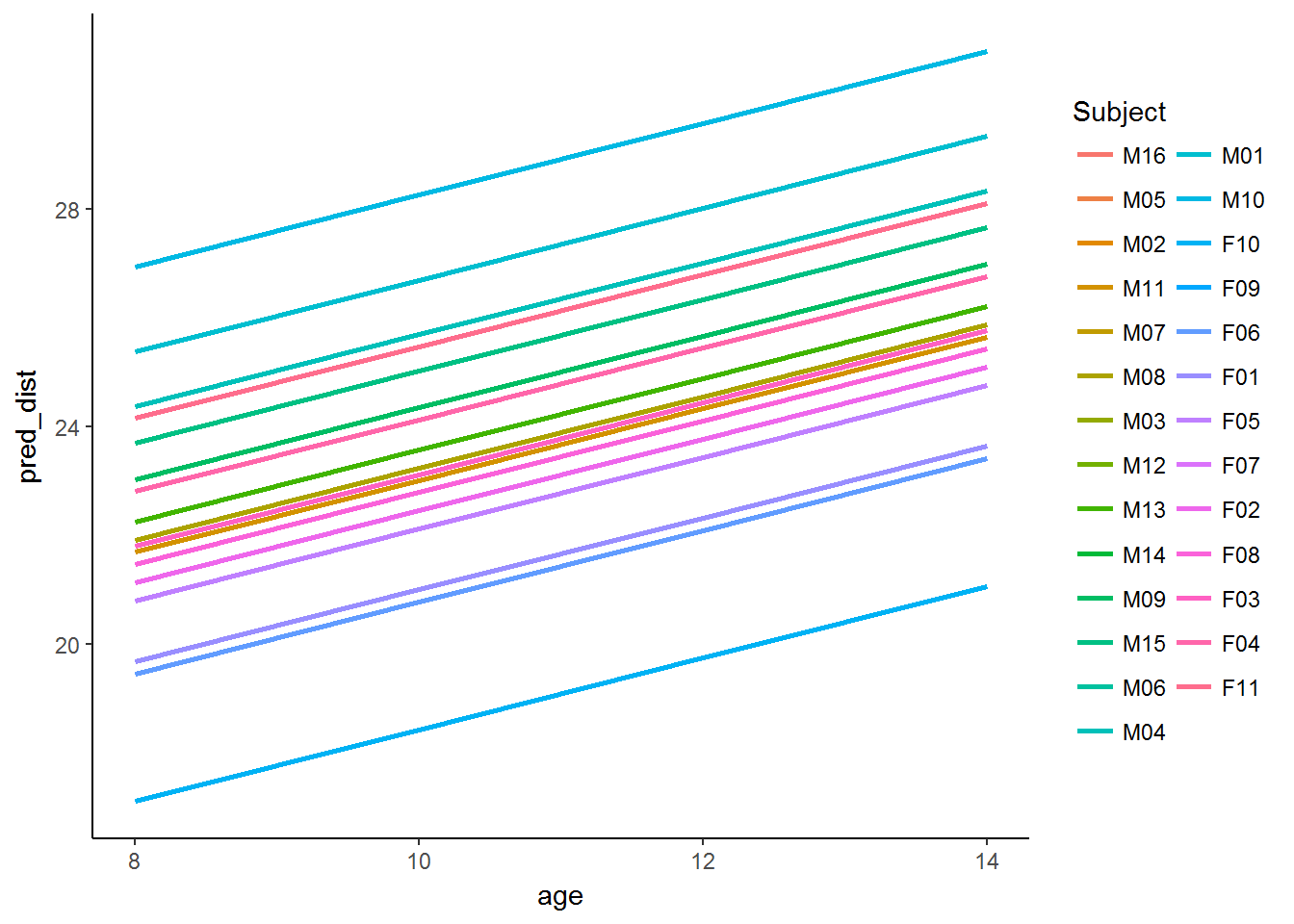## Random intercept and random slope (independent)

In this section we will allow the effect of age to vary across subjects (random slope), but we are constraining random intercept and random slope to be independent.

Level-1: $$distance_{ij}=\beta_{0j}+\beta_{1j}age_{ij}+r_{ij}$$

Level-2: $$\beta_{0j}=\gamma_{00}+u_{0j}$$

Level-2: $$\beta_{1j}=\gamma_{10}+u_{1j}$$

mod3 <- lmer(distance ~ age + (1|Subject) + (0+age|Subject), data=data1, REML=F)
summary(mod3)
## Linear mixed model fit by maximum likelihood  ['lmerMod']
## Formula: distance ~ age + (1 | Subject) + (0 + age | Subject)
##    Data: data1
##
##      AIC      BIC   logLik deviance df.resid
##    449.7    463.1   -219.9    439.7      103
##
## Scaled residuals:
##     Min      1Q  Median      3Q     Max
## -3.7542 -0.5056  0.0181  0.5216  3.8017
##
## Random effects:
##  Groups    Name        Variance Std.Dev.
##  Subject   (Intercept) 1.82570  1.3512
##  Subject.1 age         0.02141  0.1463
##  Residual              1.85944  1.3636
## Number of obs: 108, groups:  Subject, 27
##
## Fixed effects:
##             Estimate Std. Error t value
## (Intercept) 16.76111    0.70816   23.67
## age          0.66019    0.06509   10.14
##
## Correlation of Fixed Effects:
##     (Intr)
## age -0.822
data1 %>%
# save predicted values
mutate(pred_dist = fitted(mod3)) %>%
# graph
ggplot(aes(x=age, y=pred_dist, group=Subject, color=Subject)) + theme_classic() +
geom_line(size=1)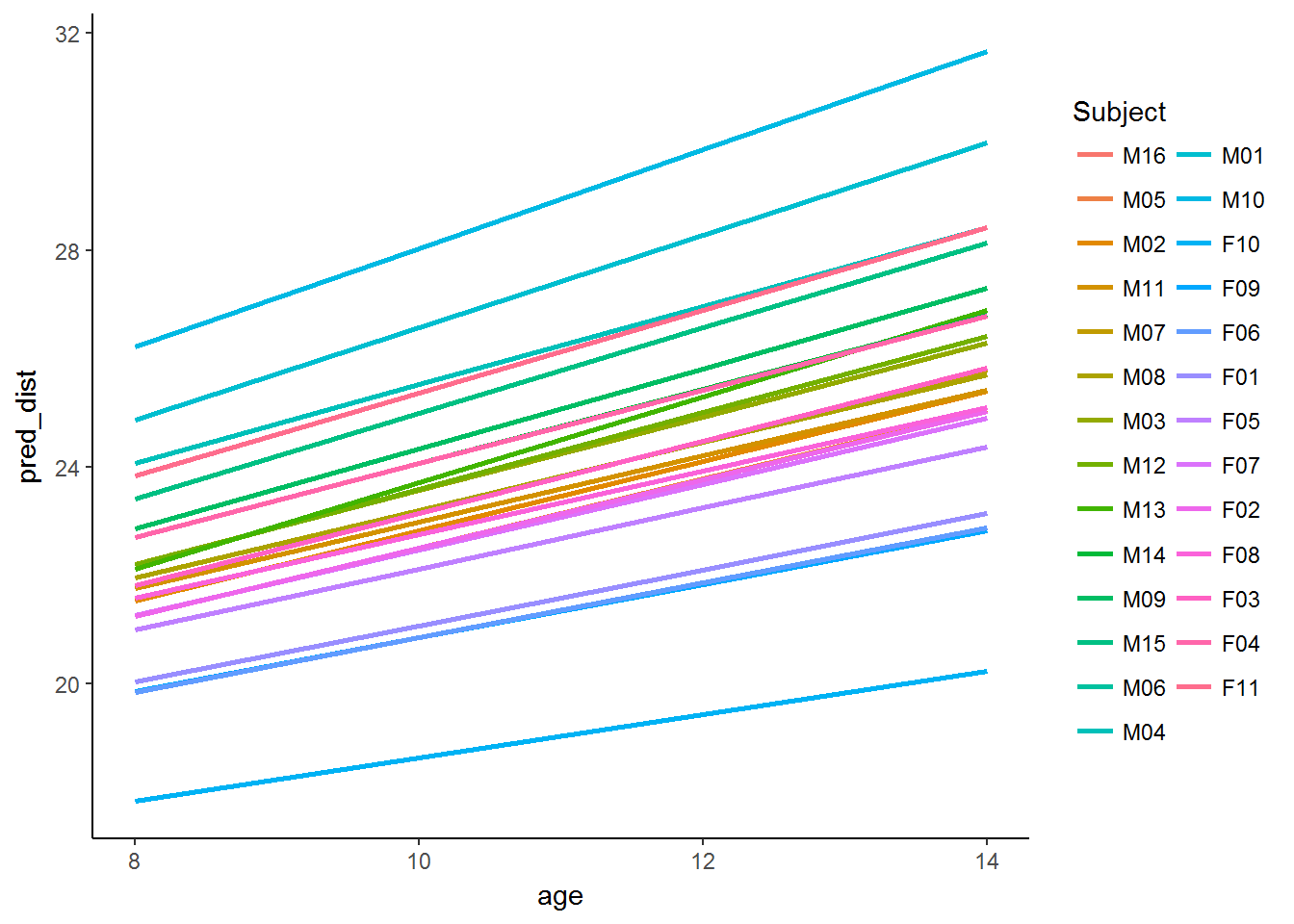## Random intercept and random slope (correlated)

This model extends the previous one by allowing random intercept and random slope to be correlated.

Level-1: $$distance_{ij}=\beta_{0j}+\beta_{1j}age_{ij}+r_{ij}$$

Level-2: $$\beta_{0j}=\gamma_{00}+u_{0j}$$

Level-2: $$\beta_{1j}=\gamma_{10}+u_{1j}$$

mod4 <- lmer(distance ~ age + (1+age|Subject), data=data1, REML=F)
summary(mod4)
## Linear mixed model fit by maximum likelihood  ['lmerMod']
## Formula: distance ~ age + (1 + age | Subject)
##    Data: data1
##
##      AIC      BIC   logLik deviance df.resid
##    451.2    467.3   -219.6    439.2      102
##
## Scaled residuals:
##     Min      1Q  Median      3Q     Max
## -3.3060 -0.4874  0.0076  0.4822  3.9228
##
## Random effects:
##  Groups   Name        Variance Std.Dev. Corr
##  Subject  (Intercept) 4.81409  2.1941
##           age         0.04619  0.2149   -0.58
##  Residual             1.71620  1.3100
## Number of obs: 108, groups:  Subject, 27
##
## Fixed effects:
##             Estimate Std. Error t value
## (Intercept) 16.76111    0.76075  22.032
## age          0.66019    0.06992   9.442
##
## Correlation of Fixed Effects:
##     (Intr)
## age -0.848
data1 %>%
# save predicted values
mutate(pred_dist = fitted(mod4)) %>%
# graph
ggplot(aes(x=age, y=pred_dist, group=Subject, color=Subject)) + theme_classic() +
geom_line(size=1)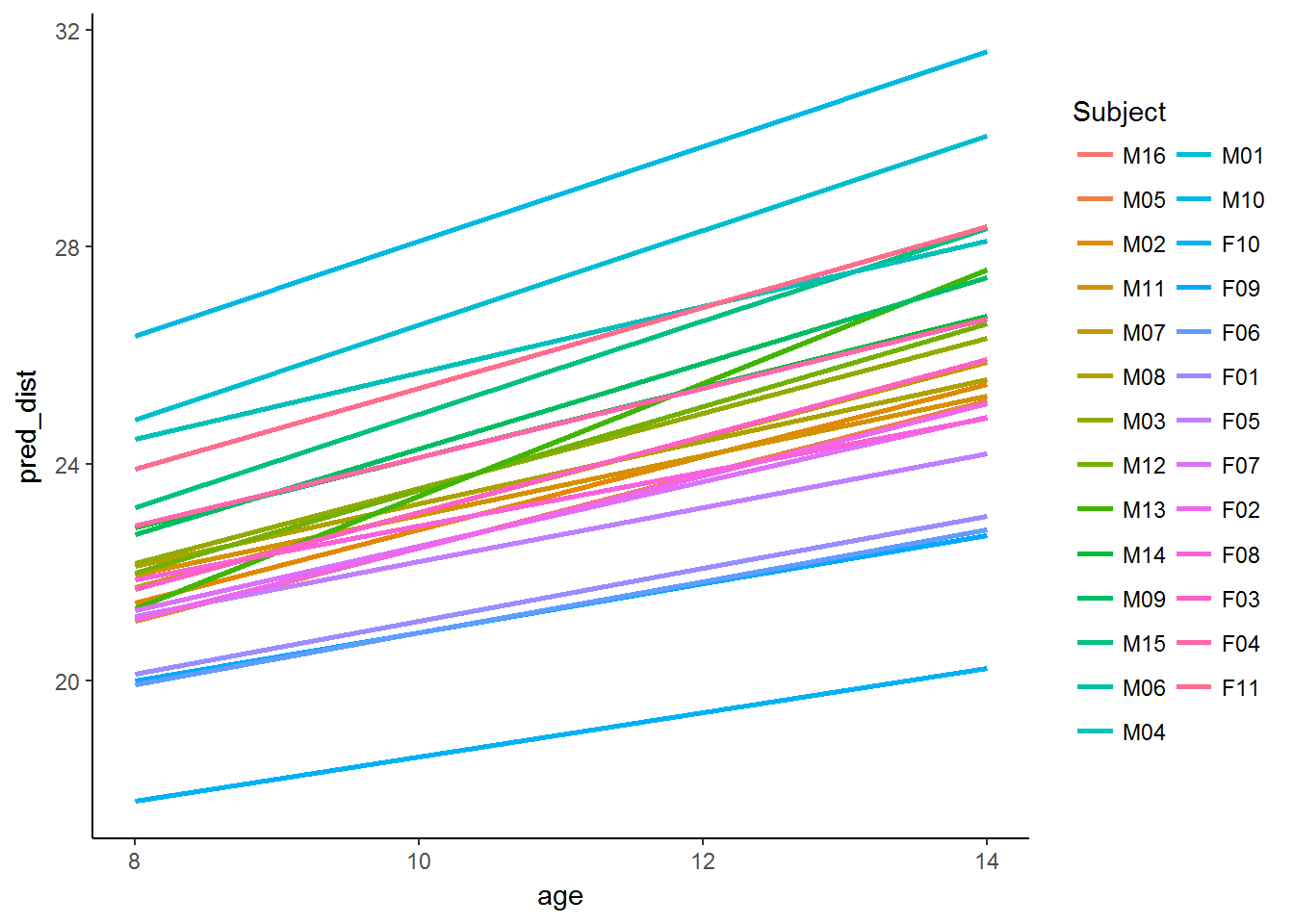## Level 1 residuals

Some graphs of the level 1 residuals.

A violin plot and a histogram:

data1 %>%
# data
mutate(L1_resid = residuals(mod4, type = "response")) %>%
# graph
ggplot(aes(x=1, y=L1_resid)) + theme_classic() +
geom_violin()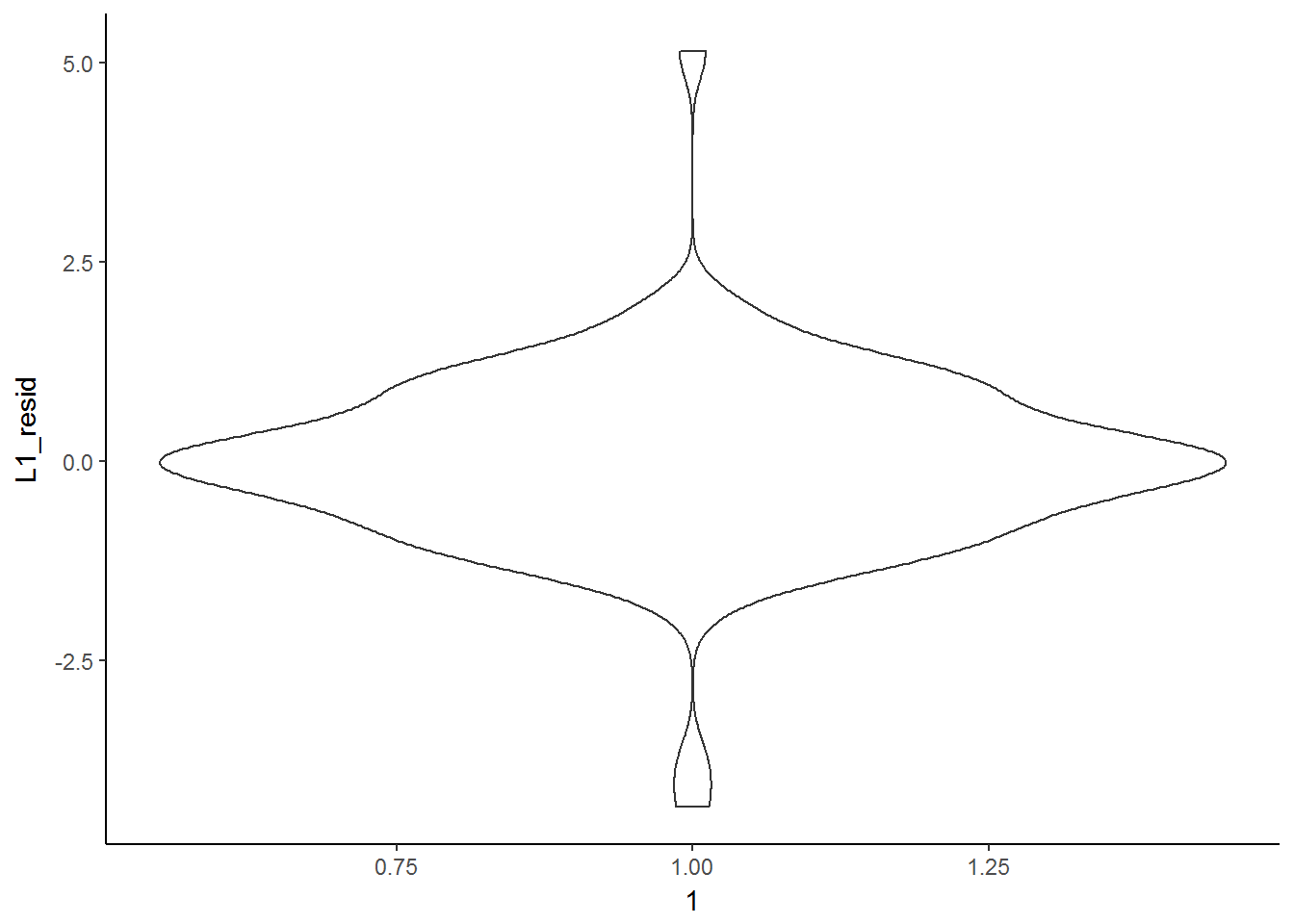data1 %>%
# data
mutate(L1_resid = residuals(mod4, type = "response")) %>%
# graph
ggplot(aes(x=L1_resid)) + theme_classic() +
geom_histogram(colour="black", fill="grey") 
## stat_bin() using bins = 30. Pick better value with binwidth.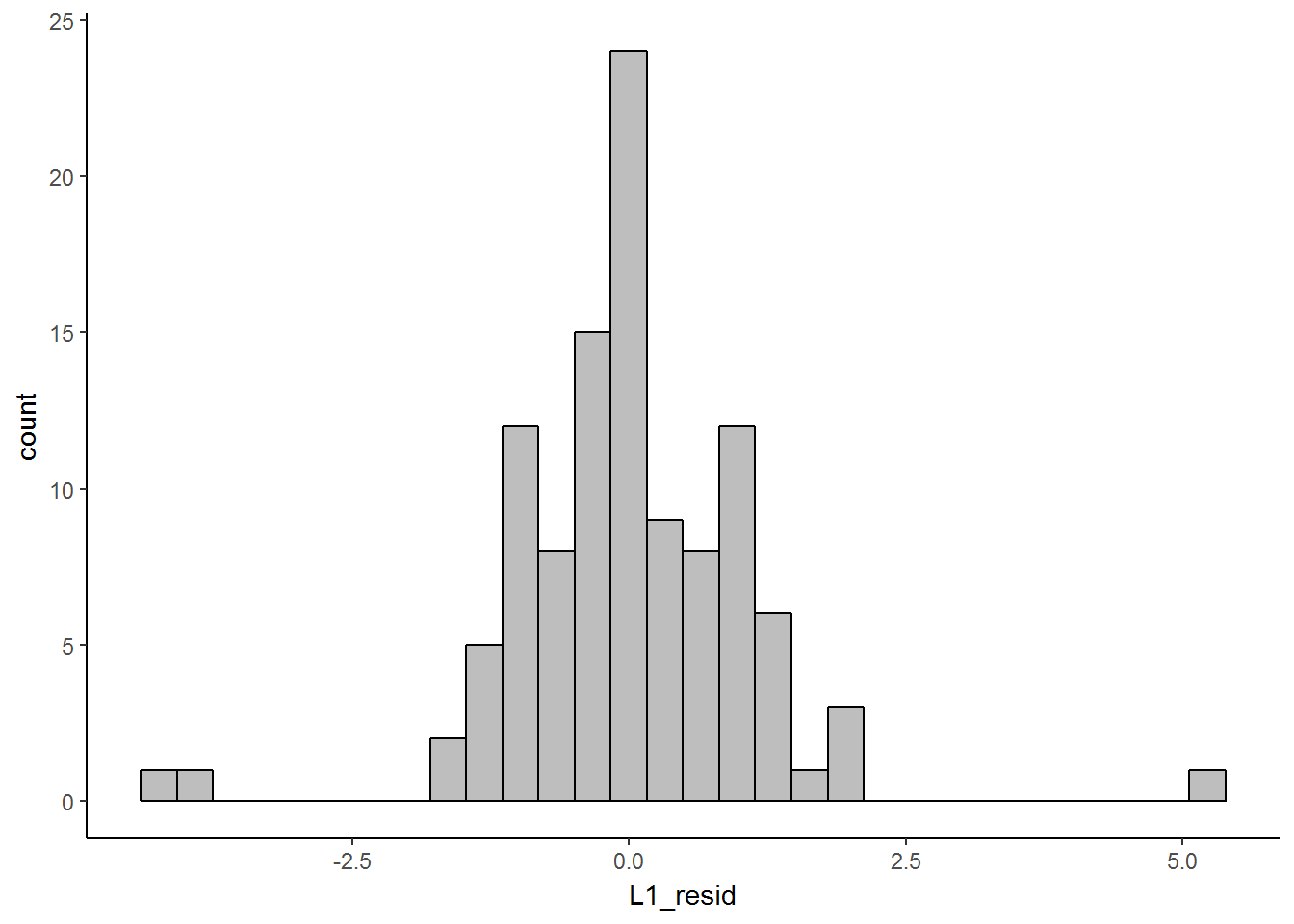Residual vs fitted plots are useful to detect non-linearity, outliers, and unequal error variances.

data1 %>%
# data
mutate(pred_dist = fitted(mod4)) %>%
mutate(L1_resid = residuals(mod4, type = "response")) %>%
# graph
ggplot(aes(x=pred_dist, y=L1_resid)) + theme_classic() +
geom_point(size=3, shape=1) +
geom_hline(yintercept=0)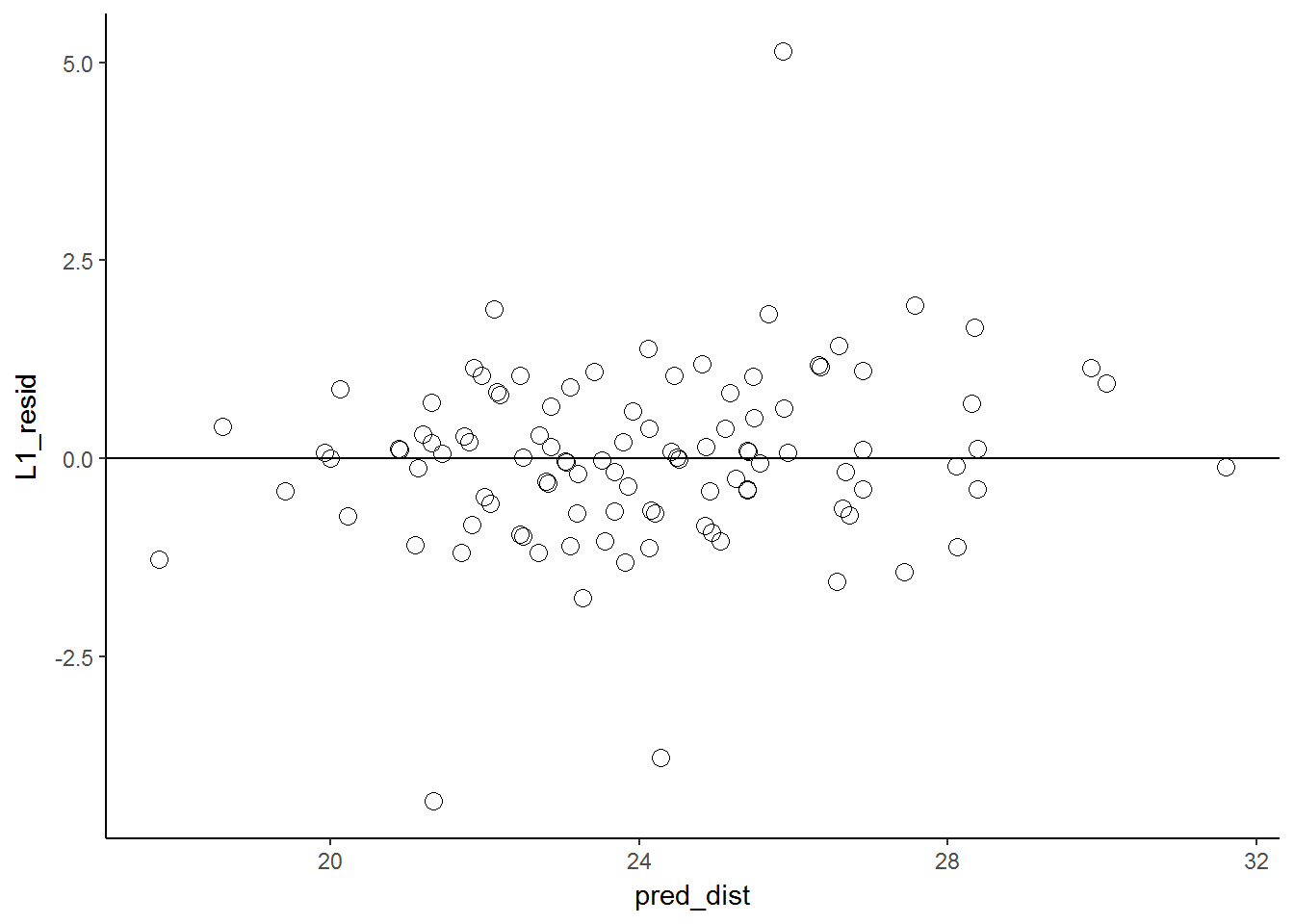## Level 2 residuals

dotplot(ranef(mod4, condVar = T), strip = T, scales=list(relation='free'))\$Subject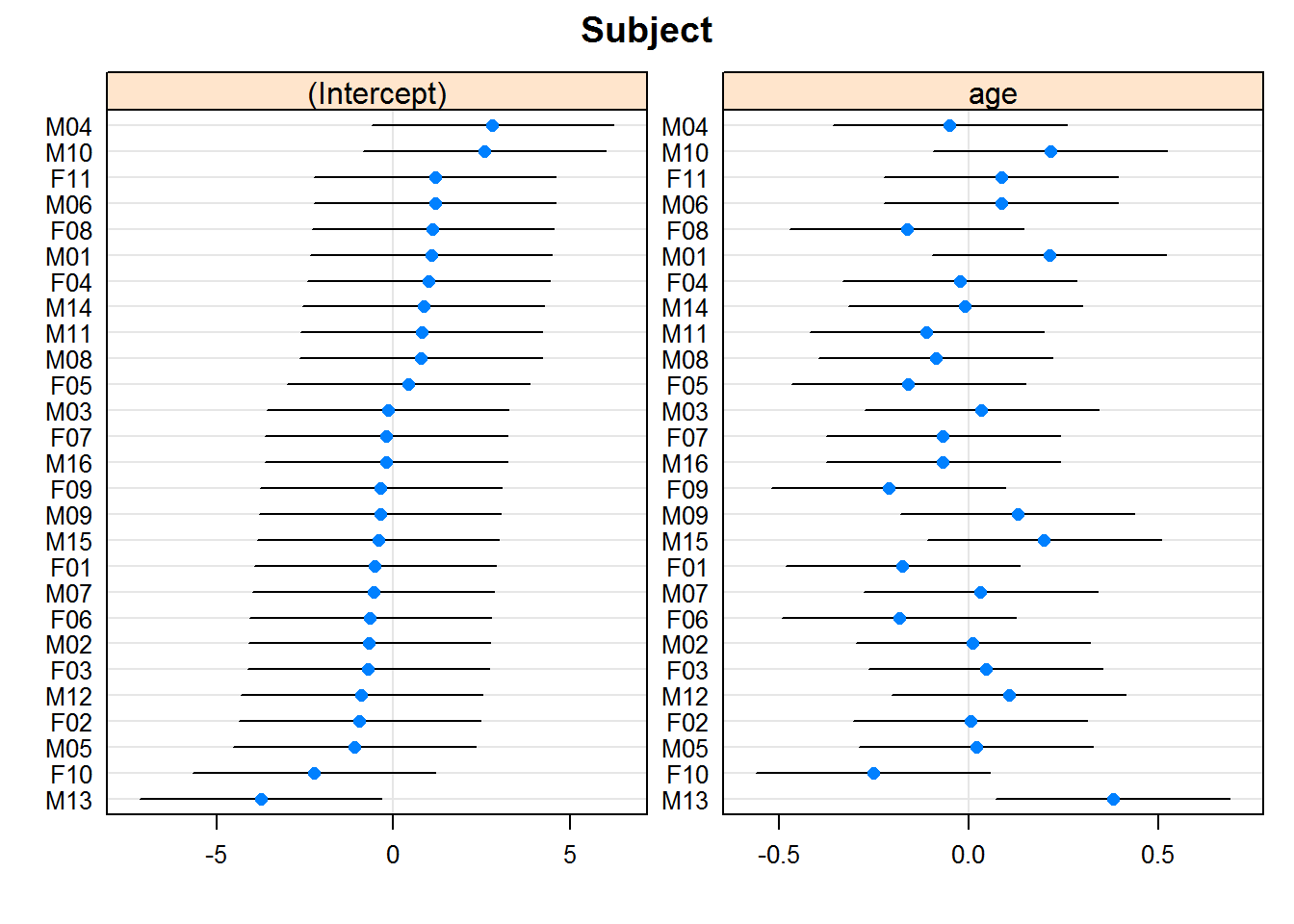## Model comparison

anova(mod1, mod2, mod3, mod4)
## Data: data1
## Models:
## mod1: distance ~ (1 | Subject)
## mod2: distance ~ age + (1 | Subject)
## mod3: distance ~ age + (1 | Subject) + (0 + age | Subject)
## mod4: distance ~ age + (1 + age | Subject)
##      Df    AIC    BIC  logLik deviance   Chisq Chi Df Pr(>Chisq)
## mod1  3 521.49 529.54 -257.75   515.49
## mod2  4 451.39 462.12 -221.69   443.39 72.1016      1    < 2e-16 ***
## mod3  5 449.74 463.15 -219.87   439.74  3.6513      1    0.05603 .
## mod4  6 451.21 467.30 -219.61   439.21  0.5267      1    0.46801
## ---
## Signif. codes:  0 '***' 0.001 '**' 0.01 '*' 0.05 '.' 0.1 ' ' 1

Categories R